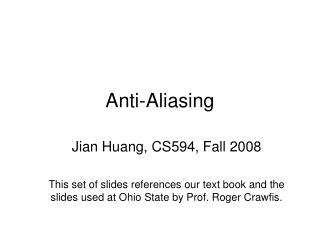DownloadDownload PresentationAnti-Aliasing

# Anti-Aliasing

Télécharger la présentation## Anti-Aliasing

- - - - - - - - - - - - - - - - - - - - - - - - - - - E N D - - - - - - - - - - - - - - - - - - - - - - - - - - -
##### Presentation Transcript

1. Anti-Aliasing Jian Huang, CS594, Fall 2008 This set of slides references our text book and the slides used at Ohio State by Prof. Roger Crawfis.

2. Aliasing?

3. Aliasing • Aliasing comes from in-adequate sampling rates of the continuous signal • The theoretical foundation of anti-aliasing has to do with frequency analysis • It’s always easier to look at 1D cases, so let’s first look at a few of those.

4. Example of Sampling

5. Examples of Inadequate Sampling

6. Cosine Integrations • Consider these formulas

7. Improper Cosine Integrals • Evaluating from (-,)

8. Fourier Transform • The Fourier Transform represents a periodic function as a continuous summation of sin’s and cos’s.

9. Fourier Transform • Note: • This is also called the direct current or DC component.

10. Inverse Fourier Transform • We can get back our original function f(x) from F(u) using the inverse transform:

11. OK – Why do this? • Fourier space is a very good space for analyzing and understanding our signals. • Rarely ever want to transform to Fourier space. • There are some great theories developed in terms of sampling and convolution.

12. Fourier Analysis • By looking at F(u), we get a feel for the “frequencies” of the signal. • We also call this frequency space. • Intuitively, you can envision, the sharper an edge, the higher the frequencies. • From a numerical analysis standpoint, the sharper the edge the greater the tangent magnitude, and hence the interpolation errors.

13. Fourier Analysis • Bandlimited • We say a function is bandlimited, if F(u)=0 for all frequencies u>c and u<-c. • Amplitude Spectrum • The magnitude, |F(u)|, is called the amplitude spectrum or simply the spectrum. • Phase Spectrum or Phase

14. Fourier Properties • Linearity • Scaling

15. Convolution • Definition:

16. Convolution • Pictorially f(x) h(x)

17. x Convolution h(t-x) f(t)

18. Convolution • Consider the function (box filter):

19. Convolution • This function windows our function f(x). f(t)

20. Convolution • This function windows our function f(x). f(t)

21. Convolution • This function windows our function f(x). f(t)

22. Convolution • This function windows our function f(x). f(t)

23. Convolution • This function windows our function f(x). f(t)

24. Convolution • This function windows our function f(x). f(t)

25. Convolution • This function windows our function f(x). f(t)

26. Convolution • This function windows our function f(x). f(t)

27. Convolution • This function windows our function f(x). f(t)

28. Convolution • This function windows our function f(x). f(t)

29. Convolution • This function windows our function f(x). f(t)

30. Convolution • This function windows our function f(x). f(t)

31. Convolution • This function windows our function f(x). f(t)

32. Convolution • This function windows our function f(x). f(t)

33. Convolution • This function windows our function f(x). f(t)

34. Convolution • This function windows our function f(x). f(t)

35. Convolution • This function windows our function f(x). f(t)

36. Convolution • This function windows our function f(x). f(t)

37. Convolution • This function windows our function f(x). f(t)

38. Convolution • This function windows our function f(x). f(t)

39. Convolution • This function windows our function f(x). f(t)

40. Convolution • This function windows our function f(x). f(t)

41. f(t) Convolution • This particular convolution smooths out some of the high frequencies in f(x). f(x)g(x)

42. Another Look At Convolution

43. Filtering and Convolution Different functions achieve different Results.

44. Impulse Function • Consider the special function (called impulse): such that,

45. Impulse and Convolution • Then, if we take the convolution of f(x) with d(x), we get: f(x)d(x) = f(x)

46. Sampling Function • A Sampling Function or Impulse Train is defined by: where T is the sample spacing. T

47. Sampling Function • The Fourier Transform of the Sampling Function is itself a sampling function. • The sample spacing is the inverse.

48. Convolution Theorem • The convolution theorem states that convolution in the spatial domain is equivalent to multiplication in the frequency domain, and vica versa.

49. Convolution Theorem • This powerful theorem can illustrate the problems with our point sampling and provide guidance on avoiding aliasing. • Consider: f(x) ST(x) f(t) T

50. S(u) Convolution Theorem • What does this look like in the Fourier domain? F(u)